# VertexMap Object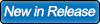The VertexMap object has undergone an extensive rewrite for this version of X-Particles and its interface has changed significantly.

Important: due to the very extensive changes to xpVertexMap over previous versions, it it not possible to import existing scenes using an xpVertexMap and retain the saved settings. Any existing xpVertexMap object will therefore revert to the default parameters with no layers added.

This object enables you to generate vertex maps from the speed of the vertices in a polygon object, or alternatively to produce the vertex map from a texture applied to the object's parent. The vertex weights in the resulting vertex map tag will be updated each frame as vertices move or as the texture changes (e.g. when using an animated texture).

Note also that it will only generate a vertex map for the object dropped into the 'Objects' list - that is, object hierarchies are not supported.

When an object is dropped into the list, it will automatically receive a vertex map tag using the name in the 'Vertex Map Name' parameter.

## Interface

The object's initial interface looks like this: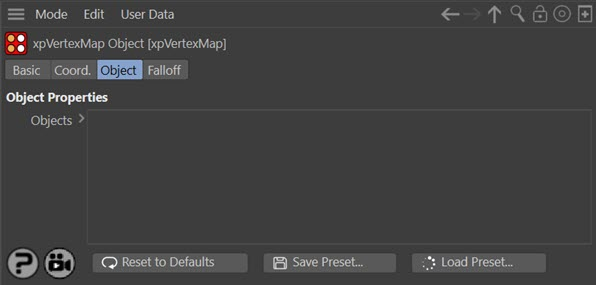For the 'Falloff' tab and for the buttons at the bottom of the interface, please see the 'Common interface elements' page.

## Parameters

Objects

Drag an object into this list. You can add multiple objects if you wish. When you drag an object, these events follow:

• the object is automatically given a vertex map tag
• a layer is added to the object and that layer appears in the list
• the layer is selected and its options are made visible

The result of adding an object to the list therefore changes the interface to look like this: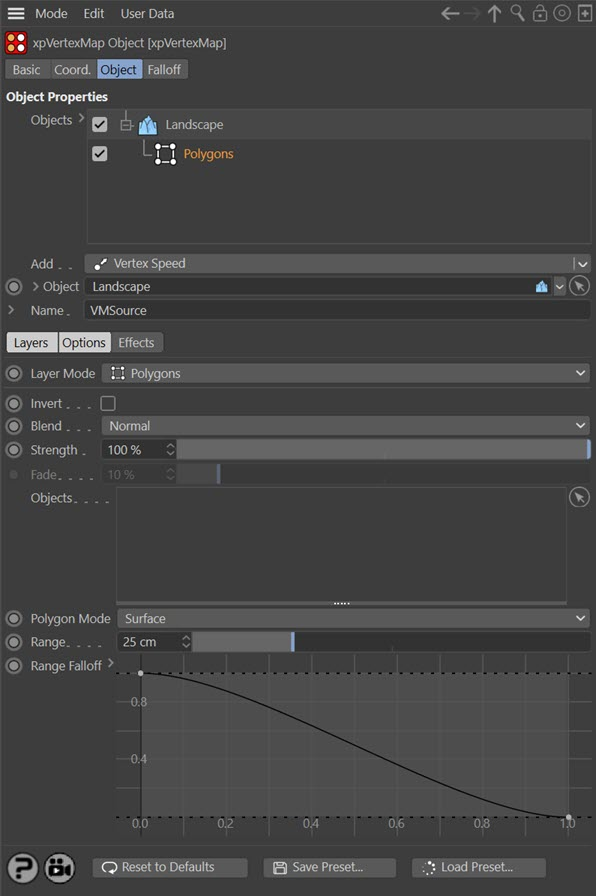You can see that the layer - which by default is a 'Polygons' layer - is selected, and there are three quicktabs, 'Layers', 'Options' and 'Effects'. of which the first two are selected and visible.

You can temporarily deactivate any object or layer in the list by unchecking the checkbox to the left of the name. To delete a layer (or an object, with all its layers), select it and press the Delete key. Alternatively, right-click the object or layer and choose 'Remove' or 'Remove All' from the context menu.

If you delete a layer, there may be none remaining for the object. Simply select the object and choose a new layer from the 'Add' menu button.

You can also reorder the layers by dragging them above or below other layers, and for blending purposes the layer order is important.

### Objects

This is a list of objects which will receive a vertex map tag. Each object may have one or more layers, as discussed below.

If you delete an object from the scene which is in the 'Objects' list, the entry is not removed from the list, but instead it reads '<Empty>' like so:You can either delete that entry and add a new one, or, with the entry selected, drag a new object into the 'Object' field below the list. This object will replace the '<Empty>' entry in the list, retaining all the layers.

This menu button enables you to add new layers to the object, which are blended with the layers below it if they are on the same object. The layer options are:

### Object

This is the currently-selected object or the object to which the currently-selected layer is attached. It is a link field, so you can drag a different object into this field and it will replace the object in the 'Objects' list.

Objects can be editable polygon objects, primitive objects, generators such as Extrude, etc, and splines. Note that the generation of vertex maps on parametric objects i not normally possible in Cinema 4D but can be done with the X-Particles VertexMap object.

### Name

This is the name of the vertex map tag that will be created. If you change it, the name of the tag on the object will be updated automatically. The default name is 'VMSource'.

You cannot delete the vertex map tag once created - or at least, you can, but it will be recreated automatically. If you really need to delete the tag, turn the Vertex Map object off in the object manager and then delete the tag.

If you click the little arrow next to the 'Name' label there are additional options in Cinema 4D R18 and higher (vertex color tags are not available in earlier versions):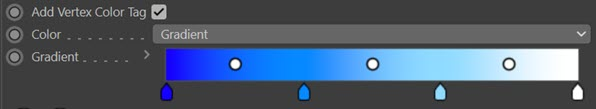Check this switch to add a vertex color tag as well as a vertex map tag. This will enable the following two options.

#### Color Mode

This is the colour mode for the tag. The options are:

The vertex colours are obtained from the 'Gradient' setting. The vertex weight determines the position along the gradient from which the colour is selected.

##### Source

Colours are obtained from the source used to generate the vertex map. For example, if an X-Particles emitter is used, the colours in the map are the particle colours. If you use a 'Polygons' layer, the colours are taken from the object colour of the object(s) used to generate the vertex map.

## Layers section

The settings in this section are common to all layers regardless of type, except for 'Fade'.

### Layer Mode

This menu is the same as the 'Add' menu below the 'Objects' list. It enables you to change from one type of layer to another without having to delete the layer and add a new one.

### Invert

If checked, the result generated by this layer is inverted.

### Blend

This is a powerful option which lets you blend layers together. Each layer is blended with the one below it in the list. Layers are evaluated from the bottom of the list upwards, so if you have three layers, layer 3 at the bottom is not blended with anything (as it has no layer below it); layer 2 is blended with layer 3 and finally layer 1 is blended with the result of blending layers 2 and 3.

When two layers are blended they use the algorithm selected from this menu, which can be different for each layer, and the blend is weighted by the 'Strength' setting in the upper layer of the two.

As an example, assume we have two layers arranged like so: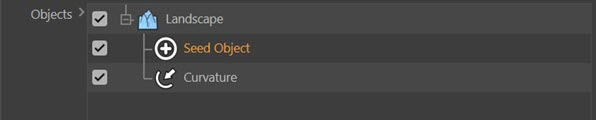What will happen if we blend these together? The layers on their own produce these results at 60 frames of animation (Seed Object layer is on the left):The blend modes are:

#### Normal

The values from the two layers are simply blended using the strength setting in the 'Seed Object' layer. If the strength is 100%, the 'Curvature' layer values make no contribution to the overall result, which is entirely determined by the 'Seed Object, values. On the other hand, if the strength is zero, the 'Seed Object' values make no contribution to the overall result, which is entirely determined by the 'Curvature' values.

#### Min

The smaller of the two values from the 'Seed Object' and 'Curvature' layers is blended with the 'Curvature' value.

#### Subtract

The 'Seed Object' value is subtracted from the 'Curvature' value and the result is blended with the 'Curvature' value.

#### Multiply

The two values are multiplied together and the result is blended with the 'Curvature' value.

#### Max

The larger of the two values from the 'Seed Object' and 'Curvature' layers is blended with the 'Curvature' value.

The two values are multiplied together and the result is blended with the 'Curvature' value.

#### Screen

This is the opposite of Multiply. The two values are inverted, multiplied together and the result is inverted again then blended with the 'Curvature' value.

Adds vertex weights then fades them. This can produce results such as this:

### Strength

This is the blend weight used to blend a layer with the layer(s) beneath it. A value of zero will always result in the upper layer making no contribution to the final value, but a value of 100% does NOT mean that only the upper layer is used for the values, except when 'Blend' is set to 'Normal'.

Only available if 'Blend' is set to 'Fade Then Add'. The higher the value, the faster the vertex weight will fade.

## Options section

Each layer type may have additional options that can be edited. These are presented here for each layer type.

### ExplosiaFX

With this option, the map is set from one of several parameters in an Explosia FX object. The map is updated as the Explosia simulation plays but resets to zero on rewinding the scene. The options are: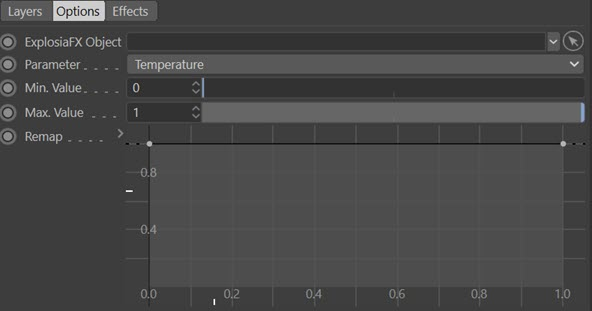As an example, here is a Figure object (made editable) and used as a source for an Explosia solver. The vertex map is generated from the temperature of voxels in the solver:

You can see the vertex map being updated as the solver proceeds (the solver itself has been hidden so you can see the vertex map clearly).

#### Explosia Object

The Explosia FX object to use. In this case the object to be weighted does not need to be an Explosia FX source object, but it must be located within the solver bounds.

#### Parameter

The Explosia parameter to use. You can choose between:

• Smoke
• Temperature
• Fire
• Velocity (X)
• Velocity (Y)
• Velocity (Z)
• Color (R)
• Color (G)
• Color (B)

#### Min. Value, Max. Value

This is the range of weight values that can be set. The defaults are 0 and 1 respectively, so the parameter from Explosia will be mapped to between those two values. If you increase the minimum value to 0.25, for example, the minimum weight which will be set is 0.25.

#### Remap

This spline can be used to remap the calculated weight value. In its default state (see the above screenshot) it has no effect. But if, for example, you lower the point at the right-hand end of the spline, so that it has a value of less than 1, this will be multiplied with the maximum calculated weight. You can animate this spline to alter the map over time.

### Seed Object

To demonstrate how this works, see this animation:

As you can see, from a seed object in the middle of the mesh, the vertex map grows over the surface. In this case, it is controlled by a noise shader which governs where the weight of the map will grow to maximum and how fast.

The parameters for this mode are as follows:Click this button to create a new X-Particles Seed Object and add it to the scene as a child object of the VertexMap Maker.

Note that without at least one seed object the vertex weights will all remain at their default and will not grow across the object.

#### Mode

There are two possible ways in which this mode can work.

##### Nearest Vertex

In this mode, the vertex map grows from one vertex to all those connected to it by an edge. This produces a smooth growth but (if the object has many vertices) it is a slow growth. Increasing the 'Search Radius' parameter will cause the change in weight to occur over larger areas of the object. If the 'Search Radius' is too small, however, no growth may occur at all, because the distance between vertices exceeds this value.

##### Reveal

Here, the vertex map is revealed as though a mask was being removed. The mask is circular and spreads from the originating vertex with a speed set in the 'Search Radius' parameter.

#### Min. Map Value, Max. Map Value

These are the minimum and maximum values the vertex weights can have. Normally they are set to a minimum of zero and maximum of 100% but you can vary these if required. The assigned weights will not range outside these limits.

#### Rate of Change

This is the speed at which the weight changes from the initial value to its final value. With the minimum and maximum map values at their defaults, and this value set to 50%, each vertex will take two frames to reach the final value.

This is only used if 'Mode' is set to 'Reveal'. When a vertex weight reaches its maximum value, it will then search for neighbouring vertices. If any of them have not started to change weight, and are within this distance of the initial vertex, they will start to change. If this value is too low there may be no vertices within that range and no change in the vertex map will occur. If it is too high, the map may change more quickly than desired since a larger number of vertices start to change.

This function will only work if 'Mode' is set to 'Nearest Vertex'.

If this value is anything other than zero, the vertex weight will start to fade once the time in 'Start Fade At' has elapsed. This will cause the weight to decrease to zero (or increase to maximum if the 'Invert' switch is checked). The higher the value in this setting, the faster the weight will fade.

This function will only work if 'Mode' is set to 'Nearest Vertex'.

This is the time after which a vertex weight will start to fade. The countdown does not begin until a vertex weight starts to grow. This setting is not available if the 'Fade From Max.' value is at zero.

#### Check Once Only

By default this option is checked. It ensures that, once a vertex has checked for any neighbouring vertices in order to start growing the vertex weight, that vertex will not search again. This speeds up the process very considerably. If it is unchecked, each vertex is checked each frame, which is time-consuming. The only reason for unchecking this option is when a mesh is animated so that vertices may become closer to one another over time and may come within the 'Search Radius' distance, or when using an animated shader

• the rate at which a vertex weight increases
• and the maximum value the weight can reach

Using a noise shader for example, the vertex weights will grow faster in areas which are brighter and the maximum weight will also be higher in those areas. Note that the final result is still influenced by the maximum and minimum map values and the rate of change.

Using a shader is not essential. If you don't use one, the values in 'Min. Map Value' and 'Max. Map Value' determine the limits on the vertex weight. It also means that each vertex weight will grow at the same rate.

#### Scale U, Scale V, Scale W

These three parameters control the size of the shader across the sample space. To put it another way, if the 'Tile' parameter is checked a bitmap will be tiled across the sample space and the number of tiles is controlled by these parameters. If 'Tile' is unchecked, or for procedural shaders, the shader is simply scaled.

#### Offset U, Offset V, Offset W

The shader will be offset by these amounts in the respective direction.

#### Mirror U, Mirror V, Mirror W

Checking these switches will mirror the shader across the respective axis.

#### Tile

If a bitmap is used, checking this switch will cause it to be tiled across the sample space. The number of tiles is controlled by the 'Scale U' and 'Scale V' settings. The switch has no effect on procedural shaders.

### Seed Map

This layer type has the same options as 'Seed Object' plus others to allow you to select a vertex map to use as the seed: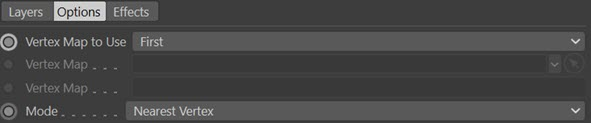#### Vertex Map to Use

From this menu you can select the vertex map to use as a seed. The options are:

##### First

The first vertex map on the object will be used as the seed.

A vertex map from the object can be dragged into the first 'Vertex Map' field and that will be used as the seed.

##### Named

Alternatively, type the name of the map to use into the second 'Vertex Map' field.

#### Vertex Map

These two fields have the same name. The first is enabled when 'Vertex Map to Use' is set to 'Linked'; it is a link field which accepts a vertex map tag. The second is enabled when 'Vertex Map to Use' is set to 'Named'; it is a text field into which you enter the name of the tag to use.

#### Mode

This menu has an additional entry - 'Clone'. If you have added another map by setting 'Vertex Map to Use' to 'Linked' or 'Named', then this option simply clones the map and will not use it as a seed - the map is simply used as it is. This enables you to add maps which have been output from other objects and perhaps combine them with additional maps generated by the Vertex Map object.

### Polygons

In this mode, the polygon face or the volume of an object is used to generate the map. In this image, a cube was used to generate a vertex map on a Landscape object. On the left, 'Polygon Mode' was set to 'Surface', on the right to 'Volume':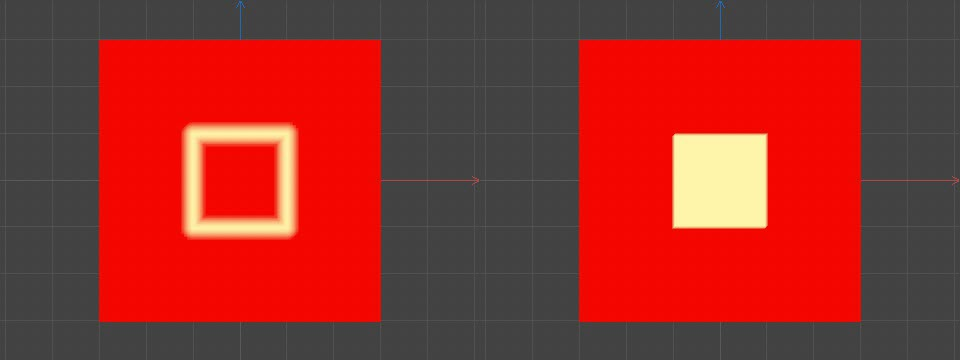You can see that in 'Surface' mode the map is generated only where the polygons of the cube touch the Landscape. When 'Volume' is used, the map is generated wherever the cube's volume intersects the Landscape.

The options are: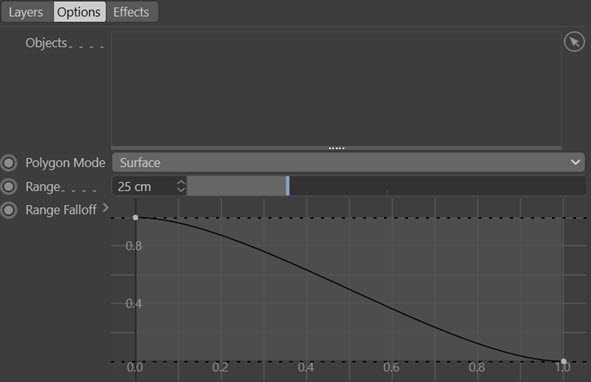#### Objects

Drag the polygon objects to use into this list.

#### Polygon Mode

Can be set to 'Surface' or 'Volume'. See the example above for details.

#### Range

Only available if 'Polygon Mode' is set to 'Surface'. A vertex must be no more than this distance away from a polygon surface for the vertex to be weighted.

#### Weight

The weight value to use if 'Mode' is set to 'Set Weight' or 'Set Weight Then Fade'.

The fade value to use if 'Mode' is set to 'Set Weight Then Fade'. The higher this value, the quicker the fade will take place.

#### Rate of Change

The value to use if 'Mode' is set to 'Increment Weight' or 'Decrement Weight'.

#### Range Falloff

If 'Polygon Mode' is set to 'Surface', this spline can be used to alter the weight value from the surface intersection. A value between 0 and 1 is obtained from the spline depending on the distance between the weighted vertex and the surface or volume. This value is then multiplied with the weight to give the final result.

With the default spline shown above, if the distance is zero (left-hand end of the spline) the full weight is used. If the distance is equal to or greater than the value in 'Range' (right-hand end of the spline) the weight or rate of change will be zero.

If 'Polygon Mode' is set to 'Volume', the 'Range' setting does not apply so only the left-hand point of the spline is used. The spline causes a uniform change in weight across all weighted vertices.

### Splines

The Splines layer is almost identical to the 'Polygons' layer, except that there is no 'Polygon Mode' setting and vertices are weighted when they are close to the the spline itself (not just its vertices). The 'Range Falloff' spline can then be used to adjust the overall weight as in the 'Polygons' layer.

See the 'X-Particles/Vertices' layer for an example of the difference between using splines in these two layers.

### Texture

This layer will generate a vertex map using a texture:#### Texture Tag to Use

##### First Tag on Object

The first texture tag on the object will be used. This is convenient if it has only one texture tag.

##### Selected Tag

You can select the desired tag by dragging it into the 'Texture Tag' field.

#### Texture Tag

Drag the texture tag you want to use from the parent object into this field. Only available when 'Texture Tag to Use' is set to 'Selected Tag'.

There are some limitations on textures:

• Only channel shaders can be used, not materials such as Hair, Nukei, etc.
• The texture must use UV projection as it is sampled by UV mapping. If you use a Noise shader, you should set its 'Space' parameter to 'UV (2D)' or what you see in the viewport will not match the rendered result, if you need to see that.

#### Texture Mode

This drop-down controls how the texture colour will be used to generated the vertex map. The default is 'Overall Brightness' which uses the colour brightness to generate the map. This means that a colour of pure red will generate the same vertex map as a pure blue colour, since they have the same brightness but different hue.

To use different colours you can select the 'Red Only', 'Green Only' or 'Blue Only' options. With these, the brightness of each colour in the sampled texture will control the vertex map weights.

#### Texture Channel

This drop-down is a list of possible texture channels to sample. You can use any of these channels, so you can use a channel which is not being used to render the material, if you like.

#### Animated Texture

Check this box to update the vertex map each frame if an animated texture is used.

### Vertex Speed

This layer uses the speed in the 3D world of the vertices of the object which holds the vertex map. It therefore requires a moving object or nothing is generated.

The vertex map weights will be generated by the speed of each vertex as it moves when the animation is played. The vertex speed is assigned a weight from 0 to 1 using a range set with the 'Min. Speed' and 'Max. Speed' parameters. If the speed is lower than the minimum, the vertex weight is zero; if it is greater than the maximum the vertex weight is 1.0. Choose the speed range carefully in order to see the maximum change in weight.

The options are: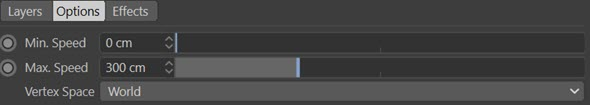#### Min. Speed, Max. Speed

The minimum and maximum speed settings for the speed range. Vertex speeds within this range will have a weight value from 0 to 1. Note that the minimum and maximum speed values are per second, not per frame.

#### Vertex Space

This drop-down lets you choose between two spaces for calculating the vertex speed. If you are moving some vertices in a mesh relative to other vertices you might want to use 'Local', and then moving the object as a whole won't affect the weight. However, if you move the entire object you need to use 'World' space to see any change in the weights. The options are:

##### Local

The vertex speed is relative to the other vertices in the object. With this setting, if you only animate the movement of the object rather than the individual vertices, the vertex weight will always be zero, since the vertices are not moving, relative to other vertices. If you animate some or all of the individual vertices their speed is calculated each frame and the vertex weight generated from that speed.

##### World

The vertex speed is relative to the object's position. This is the option to choose if you are animating an entire object (even if some vertices also move independently of the object's position and others do not).

### X-Particles/Vertices

In this layer you can use an X-Particles emitter or any object with vertices. The map will be weighted when the particle or vertex comes closer then the 'Range' value.

Note that if the object used is a spline, the map is weighted by distance from the spline's vertices, not the whole spline length. In this example, the same Star spline is used. On the left it is in an 'X-Particles/Vertices' layer, on the right in a Splines layer: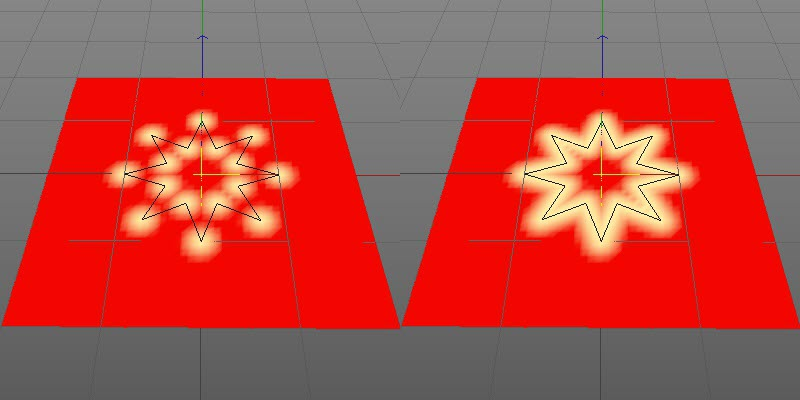The parameters are the same as the 'Polygons' layer. In this case an X-Particles emitter or any object with vertices can be dragged into the 'Objects' list. There is also this additional setting: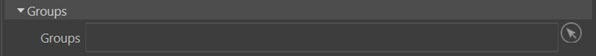### Groups

If you drag particle groups into this list, only particles in those groups are used to generate the map. If the list is empty, all particles are used.

### Curvature

The vertex map is weighted using the curvature of the object at each vertex. There are no additional options.

### Ambient Occlusion

This layer weights the vertex map depending on ambient occlusion from another object. The options are:#### Distance

The object causing ambient occlusion must be within this distance from the point being sampled. The map will become brighter the closer the occluding surface is.

#### Samples

The number of samples taken. More samples produce a more accurate result but will be slower to calculate.

#### Angle

This is the cone around the surface normal when calculating ambient occlusion. Reducing this vale will reduce the dispersion of the ambient occlsuion but may require the 'Samples' value to be increased. Compare an angle of 60 degrees: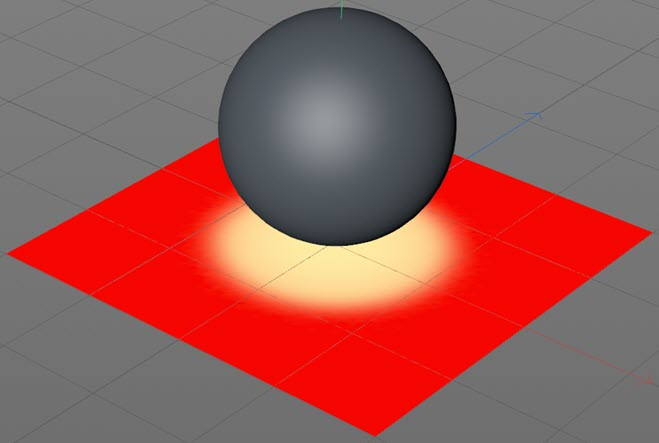With an angle of 3 degrees:Note the sharper appearance of the map when the angle is smaller.

#### Dispersion

The amount of dispersion of the vertex weights. The higher this value, the greater the spread of the vertex weights over the surface.

#### Objects

Drag any occluding objects into this list.

## Effects section

These settings are common to all layer types.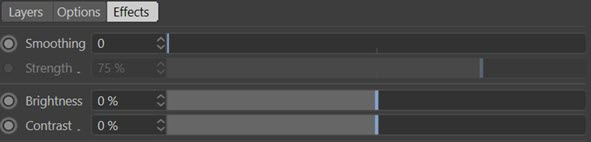### Smoothing

The number of smoothing iterations. The higher the number, the more accurate smoothing will be but it will take longer. If set to zero, there is no smoothing.

### Strength

The amount of smoothing.

### Brightness

Increase or decrease this value to increase or decrease the overall brightness of the vertex map.

### Contrast

You can alter the contrast between different weights in the map with this setting.